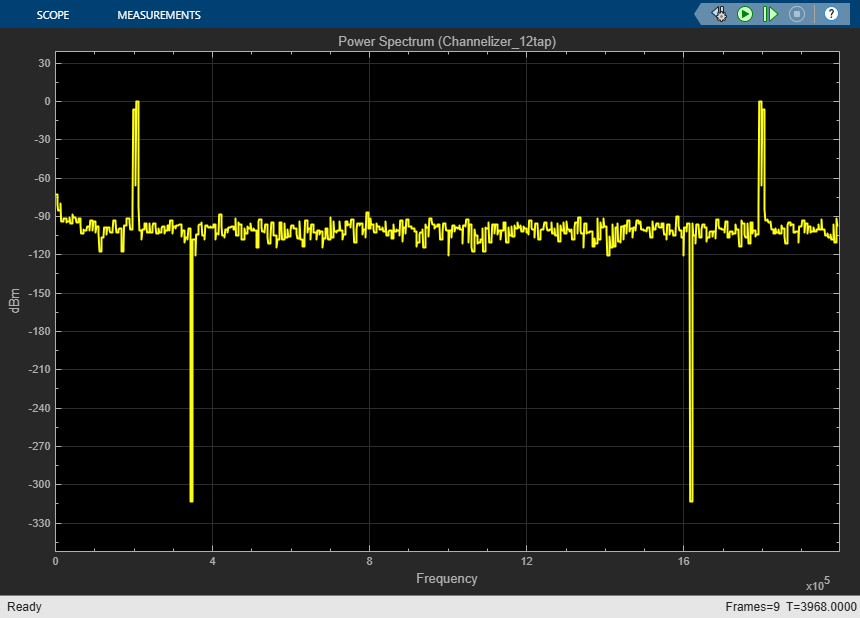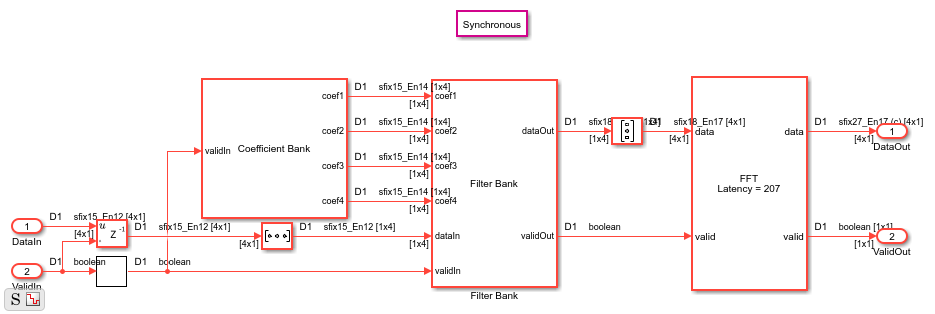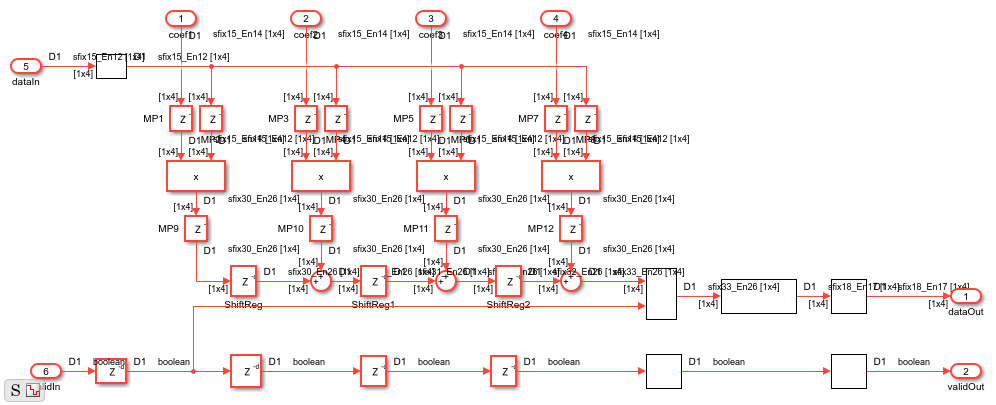# High Throughput Channelizer for FPGA

This example shows how to implement a high throughput (Gigasamples per second, GSPS) channelizer for hardware by using a polyphase filter bank.

High speed signal processing is a requirement for applications such as radar, broadband wireless and backhaul.

Modern ADCs are capable of sampling signals at sample rates up to several Gigasamples per second. But the clock speeds for the fastest FPGA fall short of this sample rate. FPGAs typically run at hundreds of MHz. An approach to perform GSPS processing on an FPGA is to move from scalar processing to vector processing and process multiple samples at the same time at a much lower clock rate. Many modern FPGAs support the JESD204B standard interface that accepts scalar input at GHz clock rate and produces a vector of samples at a lower clock rate.

In this example we show how to design a signal processing application for GSPS throughput in Simulink. Input data is vectorized through a JESD204B interface and available at a lower clock rate in the FPGA. The model is a polyphase filter bank which consists of a filter and an FFT that processes 16 samples at a time. The polyphase filter bank technique is used to minimize the FFT's inaccuracy due to leakage and scalloping loss. See High Resolution Spectral Analysis (DSP System Toolbox) for more information about the polyphase filter bank.

The first part of the example implements a polyphase filter bank with a 4-tap filter.

The second part of the example uses the Channelizer HDL Optimized block configured for a 12-tap filter. The Channelizer HDL Optimized block uses the polyphase filter bank technique.

### Polyphase Filter Bank

```modelname = 'PolyphaseFilterBankHDLExample_4tap'; open_system(modelname); ```The `InitFcn` callback (Model Properties > Callbacks > InitFcn) sets up the model. This model uses a 512-point FFT with a four tap filter for each band. Use the `dsp.HDLChannelizer` System object™ to generate the coefficients. The polyphase method of the Channelizer object generates a 512-by-4 matrix. Each row represents the coefficients for each band. The coefficients are cast into fixed-point with the same word length as the input signal.

```FFTLength = 512; h = dsp.Channelizer; h.NumTapsPerBand = 4; h.NumFrequencyBands = FFTLength; h.StopbandAttenuation = 60; coef =fi(polyphase(h),1,15,14,'RoundingMethod','Convergent'); ```

The algorithm requires 512 filters (one filter for each band). For a vector input of 16 samples we can reuse 16 filters, 32 times.

```InVect = 16; ReuseFactor = FFTLength/InVect; ```

To synthesize the filter to a higher clock rate, we pipeline the multiplier and the coefficient bank. These values are explained in the "Optimized Hardware Considerations" section.

```Multiplication_PipeLine = 2; CoefBank_PipeLine = 1; ```

### Data Source

The input data consists of two sine waves, 200 KHz and 250 KHz.

### Simulation Results

To visualize the spectrum result, open the spectrum viewers and run the model.

```open_system('PolyphaseFilterBankHDLExample_4tap/FFT Spectrum Viewer/Power Spectrum viewer (FFT)'); open_system('PolyphaseFilterBankHDLExample_4tap/PFB Spectrum Viewer/Power Spectrum viewer (PFB)'); sim(modelname); ```The polyphase filter bank Power Spectrum Viewer shows the improvement in the power spectrum and minimization of frequency leakage and scalloping compared with using only an FFT. By comparing the two spectrums, and zooming in between 100 KHz and 300 KHz, observe that the polyphase filter bank has fewer peaks over -40 dB than the classic FFT.

### Optimized Hardware Considerations

• Data type : The data word length affects both the accuracy of the result and the resources used in the hardware. For this example we design the filter at full precision. With an input data type of `fixdt(1,15,13)`, the output is `fixdt(1,18,17)`. The absolute values of the filter coefficients are all smaller than 1 so the data doesn't grow after each multiplication, and we need to add one bit for each addition. To keep the accuracy in the FFT, we need to grow one bit for each stage. This makes the twiddle factor multiplication bigger at each stage. For many FPGAs it is desirable to keep multiplication size smaller than 18x18. Since a 512 point FFT has 9 stages, the input of the FFT cannot be more than 11 bits. By exploring the filter coefficients, we observe that the first 8 binary digits of the maximum coefficient are zero, and therefore we can cast the coefficients to `fixdt(1,7,14)` instead of `fixdt(1,15,14)`. Also we observe that the maximum value of the Datatype block output inside the polyphase filter bank has 7 leading zeros after the binary point, and therefore we cast the filter output to `fixdt(1,11,17)` instead. This keeps the multiplier size inside the FFT smaller than 18-by-18 and saves hardware resources.

• Design for speed:

1. State control block: The State Control block is used in Synchronous mode to generate hardware friendly code for the delay block with enable port.

2. Minimize clock enable : The model is set to generate HDL code with the Minimize Clock Enable option turned on (In Configuration Parameters choose > HDL Code Generation > Global settings > Ports > Minimize clock enables). This option is supported when the model is single rate. Clock enable is a global signal which is not recommended for high speed designs.

3. Usage of the DSP block in FPGA: In order to map multipliers into a DSP block in the FPGA, the multipliers should be pipelined. In this example we pipeline the multipliers (2 delays before and 2 delays after) by setting Multiplication_PipeLine = 2; These pipeline registers should not have a reset. Set the reset type to none for each pipeline (right-click the Delay block and select HDL Code > HDL Block Properties > Reset Type = None).

4. Usage of ROM in FPGA: The Coefficient block inside the Coefficient Bank is a combinatorial block. In order to map this block into a ROM, add a register after the block. The delay length is set by `CoefBank_PipeLine`. Set the reset type for these delays to none (right-click the Delay block and select HDL Code > HDL Block Properties > Reset Type = None).

### Generate HDL Code and Test Bench

You must have an HDL Coder™ license to generate HDL code for this example model. Use this command to generate HDL code. systemname = 'PolyphaseFilterBankHDLExample_4tap/PolyPhaseFilterBank'; makehdl(systemname);

Use this command to generate a test bench that compares the results of an HDL simulation against the Simulink simulation behavior. makehdltb(systemname);

### Synthesis Results

The design was synthesized for Xilinx Virtex 7 (xc7vx550t-ffg1158, speed grade 3) using ISE. The design achieves a clock frequency of 499.525 MHz (before place and route). At 16 samples per clock, this translates to 8 GSPS throughput. Note that this subsystem has a high number of I/O ports and it is not suitable as a standalone design targeted to the FPGA.

### HDL Optimized Channelizer

To improve the frequency response, use a filter with more taps. The following model uses the Channelizer HDL Optimized block, configured with a 12-tap filter to improve the spectrum. Using a built-in Channelizer HDL Optimized block makes it easier to change design parameters.

```modelname = 'PolyphaseFilterBankHDLExample_HDLChannelizer'; open_system(modelname); ```The model uses workspace variables to configure the FFT and filter. In this case, the model uses a 512-point FFT and a 12-tap filter for each band. The number of coefficients for the channelizer is 512 frequency bands times 12 tap per frequency band. The `tf(h)` method generates all the coefficients.

```InVect = 16; FFTLength = 512; h = dsp.Channelizer; h.NumTapsPerBand = 12; h.NumFrequencyBands = FFTLength; h.StopbandAttenuation = 60; coef12Tap = tf(h); ```

### Data Source

The input data consists of two sine waves, 200 KHz and 206.5 KHz. The frequencies are closer to each other than the first example to illustrate the difference between a channelizer and a 4-tap filter in spectrum resolution.

### Simulation Results

To visualize the spectrum result, open the spectrum viewers and run the model.

```open_system('PolyphaseFilterBankHDLExample_HDLChannelizer/PFB_4tap Spectrum Viewer/Power Spectrum viewer (PFB_4tap)'); open_system('PolyphaseFilterBankHDLExample_HDLChannelizer/Channelizer Spectrum Viewer/Power Spectrum viewer (Channelizer_12tap)'); sim(modelname); ```The Power Spectrum Viewer for the Channelizer_12tap model shows the improvement in the power spectrum of the polyphase filter bank with 12-tap filter compared to the 4-tap filter in the previous model. Compare the spectrum results for the channelizer and 4-tap polyphase filter banks. Zoom in between 100 KHz and 300 KHz to observe that the channelizer detects only two peaks while the 4-tap polyphase filter bank detects more than 2 peaks. Two peaks is the expected result since the input signal has only two frequency components.